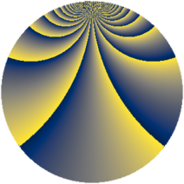# Properties

 Label 1323.2.vLevel $1323$ Weight $2$ Character orbit 1323.v Rep. character $\chi_{1323}(67,\cdot)$ Character field $\Q(\zeta_{9})$ Dimension $696$ Sturm bound $336$

# Related objects

## Defining parameters

 Level: $$N$$ $$=$$ $$1323 = 3^{3} \cdot 7^{2}$$ Weight: $$k$$ $$=$$ $$2$$ Character orbit: $$[\chi]$$ $$=$$ 1323.v (of order $$9$$ and degree $$6$$) Character conductor: $$\operatorname{cond}(\chi)$$ $$=$$ $$189$$ Character field: $$\Q(\zeta_{9})$$ Sturm bound: $$336$$

## Dimensions

The following table gives the dimensions of various subspaces of $$M_{2}(1323, [\chi])$$.

Total New Old
Modular forms 1056 744 312
Cusp forms 960 696 264
Eisenstein series 96 48 48

## Trace form

 $$696q + 3q^{2} + 3q^{3} + 3q^{4} + 3q^{5} + 18q^{6} - 12q^{8} + 27q^{9} + O(q^{10})$$ $$696q + 3q^{2} + 3q^{3} + 3q^{4} + 3q^{5} + 18q^{6} - 12q^{8} + 27q^{9} - 3q^{10} + 27q^{11} + 3q^{12} + 12q^{13} - 48q^{15} - 9q^{16} - 27q^{17} + 3q^{18} - 3q^{19} + 18q^{20} - 24q^{22} + 60q^{23} + 72q^{24} + 3q^{25} - 30q^{26} + 12q^{27} - 6q^{29} + 57q^{30} + 3q^{31} + 135q^{32} - 15q^{33} + 18q^{34} + 24q^{36} + 6q^{37} - 69q^{38} - 75q^{39} - 51q^{40} - 24q^{43} + 6q^{44} + 21q^{45} + 6q^{46} + 21q^{47} - 90q^{48} - 9q^{50} + 21q^{51} - 9q^{52} - 9q^{53} + 9q^{54} + 24q^{55} - 30q^{57} + 3q^{58} - 27q^{59} + 123q^{60} + 21q^{61} - 75q^{62} - 276q^{64} + 156q^{65} + 3q^{66} + 3q^{67} + 30q^{68} + 6q^{69} + 12q^{71} - 261q^{72} + 42q^{73} - 165q^{74} + 45q^{75} + 24q^{76} + 30q^{78} - 33q^{79} - 102q^{80} + 171q^{81} + 6q^{82} + 42q^{83} + 63q^{85} + 129q^{86} - 75q^{87} - 21q^{88} - 75q^{89} + 39q^{90} + 102q^{92} - 93q^{93} - 33q^{94} + 51q^{95} + 171q^{96} + 12q^{97} - 48q^{99} + O(q^{100})$$

## Decomposition of $$S_{2}^{\mathrm{new}}(1323, [\chi])$$ into newform subspaces

The newforms in this space have not yet been added to the LMFDB.

## Decomposition of $$S_{2}^{\mathrm{old}}(1323, [\chi])$$ into lower level spaces

$$S_{2}^{\mathrm{old}}(1323, [\chi]) \cong$$ $$S_{2}^{\mathrm{new}}(189, [\chi])$$$$^{\oplus 2}$$